# Nitrous

Nitrous[footnote: It is named for nitrous oxide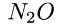, a gas used to make cars go really fast. It is also a common medical anaesthetic. William James found that its subjective effect was to reveal the Hegelian synthesis [James1882].] is a compiler generation system. It accepts a program in a full-featured source language and transforms the program into a compiler. Because it runs very slowly and the constant propagation is aggressive, excessively large compilers and residual code is a problem. The objective of the design was to demonstrate that enough static information is available to make transformations like those of Section application and Chapter bit-addr work. For example, one might wonder if higher-order functions or safety conflicts with late normalization of partially static integers or early equality.

`Root` is the intermediate language at the center of the system, it is described below. Figure system-block identifies the three kinds of program transformations within Nitrous:

• Front-ends which produce `root` programs from programs in user-defined languages. Though the front-ends discussed here are all automatically generated by the system, the design is meant to support hand-written front-ends, such as an ordinary ML compiler.

• A specializer for an untyped intermediate language `root`.

• A back-end provides machine-code assembly, optimization, and the rest of the run-time system.

The specializer is a compiler generator `cogen`. This chapter explains the mechanics of how `cogen` works, including the intermediate language and some important front-ends.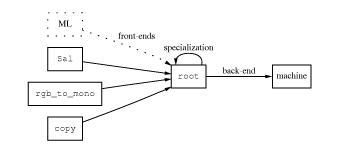ps

Figure system-block: Diagram showing components of the Nitrous system. Boxes denote languages, and arrows denote program transformations. The ML front-end is hypothetical, and so is drawn with dotted lines.

`Root` is a simple abstract machine code, like higher-order three-address-code ([ASeUl86], p. 466) with an unlimited number of registers and in continuation-passing closure-passing style (CPS-CPS) [Appel92]. Thus the stack in a `root` program is explicitly represented as a data structure. The model includes data structures, arithmetic, an open set of primitive functions, and represents higher-order values with closures.

```
```

A language supports reflection if it can convert a data structure representing an arbitrary program text into a value (possibly a function). Lisp supports reflection with `eval` and `compile`. Reification is the opposite of reflection, that is, converting a value (possibly a function) into a corresponding text. Although reification is commonly available in debuggers and interpreters, not even Common LISP standardizes it. [FriWa84] defines and discusses these ideas in detail. Reification is sometimes called introspection [Thiemann96] Section 4.

`Root` supports both reflection and reification. By supporting reflection we make code-producing functions first class. Nitrous takes this a step further by using reification to make the compiler-producing function first class: rather than working with files, cogen just maps procedures to procedures.

Sal is a recursive-equations language augmented with `lambda` and various convenience features. The `cogen`-created compiler produces straight-forward code. It performs CPS-CPS conversion and compiles tail-recursive calls without building stack frames, but is otherwise non-optimizing. Sal is covered in Section sal.

`Rgb_to_mono` (from Figure rgbm) and `copy` (from Figure sig) are two example media processing languages. The interpreters for these languages were written in Sal.

Nitrous implements specialization in two stages: `cogen` transforms a `root` program and binding times (BTs) for its arguments into a generating extension. The extension is executed with the static values to produce the specialized residual program. The BTs categorize each argument as static program or dynamic data. The extension consists of a memo table, followed by the static parts of the computation interleaved with instructions that generate residual code (i.e. do RTCG).

Because the compilers produce the same language that `cogen` accepts, and the `root` text of the residual programs is easily accessible, multiple layers of interpretation can be removed by multiply applying `cogen`. For example the output of the Sal compiler is valid input to `cogen`. This is how `rgb_to_mono` and `copy` were built. The lift compiler (see Section structlift) also uses two layers. Another possibility is to include a compiler generator as a primitive in Sal.

Multi-stage application requires that the generated compilers create correctly annotated programs, which can be difficult. In [GluJo94, GluJo95] Glück and Jørgensen present more rigourous and automatic treatments of layered systems using specializer projections and multi-stage binding-time analysis.

This chapter is organized as follows. First, the intermediate language is presented, followed by the compiler generator, the Sal front end, and the conclusion. The bulk of the material consists of technical details of the compiler generator.

## The Intermediate Language

The core of the system is the intermediate language. Its formal syntax appears in Figure root-syntax. A program is called a code pointer, or just a `code`. When a `code` is invoked, its formal parameter list is bound to the actual arguments. The list of `prim` and `const` instructions execute sequentially, each binding a new variable. `If` tests a variable and follows one of two instruction lists. These lists terminate with a `jump` or `exit` instruction. A `jump` invokes the `code` bound to the first argument. The remaining arguments form the parameter list, and the cycle repeats itself. `Exit` stops the machine and returns an answer. Formal semantics appear in Figure root-semantics. The semantics are given in Scheme [R4RS], extended with a pattern-matching macro.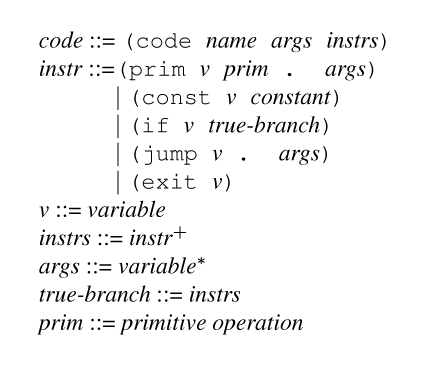Figure root-syntax: `Root` syntax. As usual, * and + superscripts denote lists of length zero-or-more and one-or-more, respectively.

```(define (eval-root code args)
(match code
(('code name formal-args source-code)
(let loop ((instrs source-code)
(env (zip formal-args args)))
(let ((lu (lookup env)))
(match instrs ; not just the car
((('exit arg)) (lu arg))
((('jump fn . args))
(eval-root (lu fn) (map lu args)))
((('if pred t-branch) . f-branch)
(if (lu pred)
(loop t-branch env)
(loop f-branch env)))
((('const var c) . rest)
(loop rest (cons (cons var c) env)))
((('prim var prim . args) . rest)
(let ((v (apply prim (map lu args))))
(loop rest (cons (cons var v) env))))))))))
```

Figure root-semantics: Semantics of `root` in Scheme.

Structured higher-order control flow is managed with closure-passing [Appel92]. A closure is a pair consisting of a code pointer with its bound variables, and is invoked by jumping to its left member and passing itself as the first argument. A normal procedure call passes the stack as the next argument. The stack is just the continuation, which is represented with a closure.

See Figure append-eg for an example program in `root` that uses a stack. Notes: a return by jumping to the car of `k`, passing `k` and `m` as arguments. b These constants are codes not symbols (`append` is a circular reference). c `close` is like `cons`, but identifies a closure. Figure app2-eg shows the same program in the abbreviated syntax used in the remaining examples. d destructuring assignment expands into car/cdr chain. Figure aper shows how to evaluate this program. Figure ho-eg shows a higher-order program.

```(code append (k l m)
((prim t0 null? l)
(if t0 ((prim t1 car k)
(jump t1 k m)))    ; a
(prim frame list k l m)
(const cont cont)          ; b
(prim cl close cont frame) ; c
(prim t2 cdr l)
(const append append)      ; b
(jump append cl t2 m)))

(code cont (self r)
((prim t0 cdr self)
(prim k car self)
(prim t1 cdr t0)
(prim l car t1)
(prim t2 cdr t1)
(prim m car t2)
(prim t3 car l)
(prim nr cons t3 r)
(prim t4 car k)
(jump k nr)))
```

Figure append-eg: `Root` code for `append`, in literal syntax. See text for notes.

```append(k l m) {
if (null? l) (car k)(k m)    ; a
frame = (list k l m)
cl = (close cont frame)      ; b c
append(cl (cdr l) m)         ; b
}
cont(self r) {
(k l m) = (cdr self)         ; d
nr = (cons (car l) r)
(car k)(k nr)
}```

Figure app2-eg: `Root` code for `append`, in sugary syntax. See text for notes.

```compose(k f g) {
lam = (close apply_g (list f g))
(car k)(k lam)
}
apply_g(k self x) {
(f g) = (cdr self)
cl = (close cont (list k f g))
(car g)(g cl x)
}
cont(self r) {
(k f g) = (cdr self)
(car f)(f k r)
}```

Figure ho-eg: `Root` code for `compose`, showing how closures and higher-order calls work.

```(define top-cont '((code top-cont (self r) ((exit r)))))

(eval-root append-root (list top-cont '(1 2 3) '(4 5)))

-->

(1 2 3 4 5)```

Figure aper: Session with Nitrous showing execution of the program `append` (which is bound to the Scheme variable `append-root` to avoid conflict with the native version). `Top-cont` is a stub continuation that returns a value to the Scheme metalevel.

Factors that weigh in favor of an intermediate language like `root` instead of a user-level language like Scheme or SML: it makes `cogen` smaller and easier to write; it provides a target for a range of source languages; it provides an interface for portability; it exposes language mechanism (such as complex optional arguments, pattern matching, method lookup, or multi-dimensional arrays) to partial evaluation; it reduces assembly overhead because it is essentially an abstract RISC code.

Two factors that weigh against `root`: explicit types would simplify the implementation and formalization, and on some architectures good loops (e.g. PC-relative addressing or other special instructions) are difficult to produce. Using a language like the JVM [GoJoSte96], or one of the intermediate languages of [TMCSHL96] would leverage existing research.

## The Compiler Generator

`Cogen` is directly implemented (rather than produced by self-application), polyvariant in binding times (it allows multiple binding time patterns per source procedure), performs polyvariant specialization with memo-tables, and handles higher-order control flow. This section summarizes how `cogen` and its extensions work. The subsections cover the implementation of binding times, cyclic integers, lifting, static extensions, special primitive functions, shape un/lifting, and dynamic control stacks.

While Nitrous does very well with small examples, several flaws make scaling difficult. Nitrous is apt to classify too much computation as static and produce large amounts of residual code, thus requiring the programmer to make careful use of lifting. Several expediencies made its developement easier, but increased the code output. Section simple describes an implementation that scales better by using a restricted language.

One interesting feature of Nitrous is that the input language is in continuation-passing style. This has three results: let-insertion for safety is avoided, dynamic choice of static values is supported, and the generated compilers do CPS transformation.

Although the input language is not explicitly typed, it turns out that annotations marking particular values as stacks and closures are essential. This indicates that an explicitly typed input language would work better.

`Cogen` converts a `code` and a binding-time pattern to a metastatic extension. A metastatic extension is denoted with the name of the code pointer and the BT pattern, for example ```append(D S D)```. The extension takes the static values and the names of the dynamic values and returns a procedure---the specialized code. Figure apsh shows an example session.

```(define append-DSD (cogen append-root
'(dynamic static dynamic)))

(eval-root append-DSD
`(,top-cont
(1 2 3)
,(var->shape 'k)
,(var->shape 'm)))

-->

append-123(k m) {
(car k)(k (cons 1 (cons 2 (cons 3 m))))
}```

Figure apsh: Session with Nitrous showing generation and execution of an extension.

```
```

Inlining is controlled by the dynamic conditional heuristic (see Section practical), but setting the special `\$inline` variable overrides the heuristic at the next jump (see Figures fdts and do-call for examples).

In CPS-CPS continuations appear as arguments, so static contexts are naturally propagated. Figure dyn-if shows the translation of `(+ s (if d 2 3))` into `root` and the result of specialization. This is the effect refered to in Section features.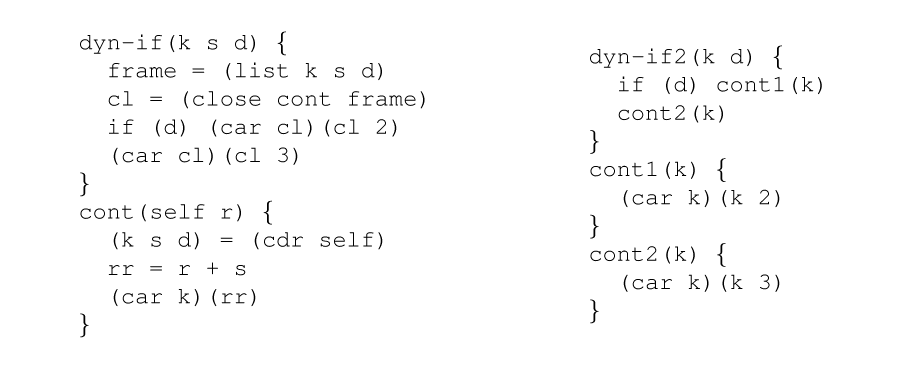Figure dyn-if: Propagating a static context past a dynamic conditional. The source code is on the left, and the residual code on the right.

### Binding Times

Binding times are properties derived from the interpreter text while a compiler generator runs. In the formal system of Chapter pe, they were the injection tags on values in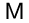. Primarily they indicate if a value will be known at compile time or at run-time, but they are often combined with the results of type inference, control flow analysis, or other static analyses. Binding times are sometimes called metastatic values because in self-application of a specializer, they are static at the meta-level (the outer application).

`Cogen`'s binding times are described by the grammar in Figure lo. The binding times form a lattice where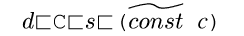and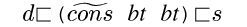. Thus `D` is the bottom of the lattice, though this contradicts the metaphore suggested by ``lifting''. Circular binding-times are allowed, so binding-times may have infinite size as long as they are recognized by a context-free grammar. The join operation of the lattice is not used by Nitrous, but the following equations define it for finite binding-times: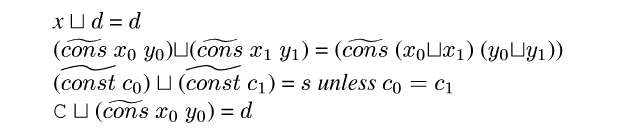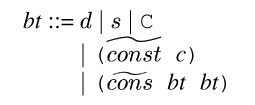S -> C -> D; S -> cons -> D; }}">
Figure lo: The binding time lattice. In the grammar on the left `D` denotes dynamic, `S` denotes static, `C` denotes cyclic, and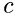denotes a constant. The lattice order is illustrated on the right.

Cons cells are handled by representing binding times with graph-grammars as Mogensen did. Pairs in binding times are labeled with a cons point that identifies which instruction the pair came from. If the same label appears on a pair and a descendant of that pair then the graph-grammar is collapsed, perhaps forming a circularity [Mogensen89]. Figures collapse and colla2 show two possibilities.

The function of the cons-points would be better provided by explicit inductive types.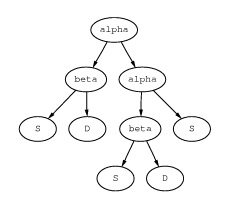ps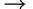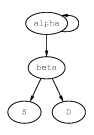ps

Figure collapse: The Cons-point `alpha` appears twice and the graph is collapsed, forming a circularity.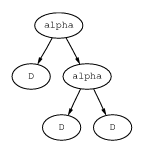ps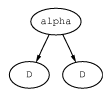ps

Figure colla2: The Cons-point `alpha` appears twice and the graph is collapsed, not forming a circularity.

We denote such a pair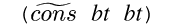(the label is invisible here).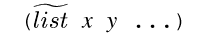abbreviates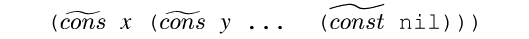. We use familiar type constructors to denote circular binding times. Figure bt-eg depicts several useful examples.

As in Schism [Consel90], control-flow information appears in the binding times. `Cogen` supports arbitrary values in the binding times, including `code` pointers, the empty list, and other type tags. Such a binding time is denoted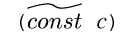, or just c.

Closures are differentiated from ordinary pairs in the `root` text, and this distinction is maintained with a bit in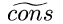binding times. Such a binding time is denoted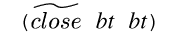.

An additional bit on pair binding times supports a sum-type with atoms. In terms of a grammar, awith this bit set may be either a pair or an atom (a terminal). The bit is set during collapse if a cons is summed with an atom; in Figure collapse the bit is set on `alpha`. In Figure colla2, the bit is not set. The bit is not denoted.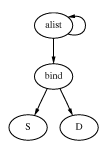ps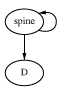ps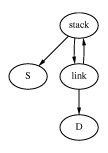ps

Figure bt-eg: Three binding times: `(S * D) list` is typically used as an association list from static keys to dynamic values, `D list` is a list only whose length is static, stack-bt is the binding time of a control stack.

Nitrous can break an integer into static base, dynamic quotient, and static remainder, as described in Section cyclic. In the lattice, `S`<`C`<`D`. We have to make special cases of all the primitives that handle cyclic values; these were introduced in Section cyclic. See Section prims for a description of the other special primitives.

### Shapes and Sharing

Extensions rename the variables in the residual code and keep track of the shapes (names and cons structure) of the dynamic values. The shape of a simple dynamic value is the name of the variable that will hold the value. If the shape of a value is a pair of names, then the dynamic value is held in two variables. Shapes always mirror the binding times. In partial evaluation jargon, the effect of using shapes is called variable splitting or arity raising.

In Figure apsh, the binding time of the third argument is `D`, so its shape must be just a name. In Figure apsh2, the binding time is `D list`, so the shape is a list of names. In Figure apsh3 the binding time is the same, but because Nitrous uses the sharing information from the identical names in the shape, only two values are passed instead of four.

Shapes are part of the key in the static memo-table. Two shapes match only if they have the same aliasing pattern, that is, not only do the structures have to be the same, but the sharing between parts of the structures must be the same. This can be computed in nearly-linear time.

The effect of variable splitting is that the members of a structure can be kept in several registers, instead of allocated on the heap (abstracted into one register). The effect of sharing is that if two of the members are known to be the same, they only occupy one register. This comes down to adding equational constraints to the static information. This is why the residual code from `rgb_to_mono` in Figure rgbm1 has fewer arguments than Figure rgbm2.

```(define append-DSD*
(cogen append-root
`(dynamic static ,(make-list-bt 'dynamic))))

(eval-root append-DSD*
`(,top-cont
(2 3)
,(var->shape 'k)
,(map var->shape '(a b c))))
-->
append-23abc(k a b c) {
t = (cons a (cons b (cons c '())))
(car k)(k (cons 2 (cons 3 t)))
}```

Figure apsh2: Session with Nitrous showing the effect of shapes. `Make-list-bt` creates `D list` from `D`.

```(eval-root append-DSD*
`(,top-cont
(2 3)
,(var->shape 'k)
,(map var->shape '(a b b a))))
-->
append-23abba(k a b) {
t = (cons a (cons b (cons b (cons a '()))))
(car k)(k (cons 2 (cons 3 t)))
}```

Figure apsh3: Session with Nitrous showing the effect of sharing.

### Lifting

Lifting is generalization, or abstracting away information. If we abstract away the right information the compiler will find a match in its memo table, thus proving an inductive theorem and forming a dynamic loop. The simplest lift converts a known value to an unknown value in a known location (virtual machine register). Lifting occurs when

• a metastatic `code` is converted to a static value.

• a `prim` has arguments of mixed binding time, causing all the arguments to be lifted.

• a `jump` has dynamic target, causing all the arguments to be lifted.

• a Cons-point repeats in a binding-time grammar, causing its collapse (Figures collapse and colla2).

• a lift directive appears, as a result of manual annotation, or produced by a delayed lift.

Lifting is inductively defined on the binding times. The base cases are:

1.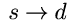allocates a dynamic location and initializes it to the static value.

2.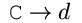emits a multiplication by the base (unless it is one) and addition with the remainder (unless it is zero).

3.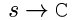results from an annotation used to introduce a cyclic value. The conversion is underconstrained; currently a base of one is assumed. This has been obviated by `set-base`.

4.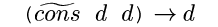emits a dynamic `cons` instruction.

5.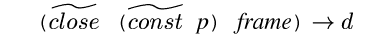generates and inserts a call to `p((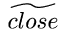D x) D D ...)` (all but the first argument are `D`), then emits the cons pairing the specialized code with the dynamic values.

6.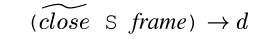This is lifting a static extension to dynamic, so it is like the previous case, but the binding times are passed to the extension.

Cases 5 and 6 are particularly interesting. Any static information in frame is saved by reassociating it into the code pointer before it is lifted. This introduces a complication, as explained in Section unlift below.

```
```

Manual lifting is supported in `root` with an instruction understood by `cogen` but ignored by the `root` semantics: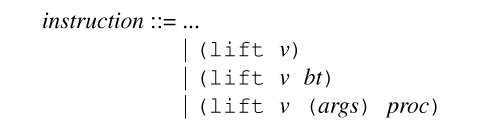The variable v is lifted to `D`, unless the target bt is given. Any legal lift is supported, including lifting to/from partially static structures with loops and closures. Instead of giving a binding time bt, one can give a procedure proc which is executed on the binding times of args. This provides a gateway to Scheme for the lift language.

A delayed lift, denoted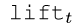, produces a residual lift at time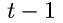. If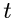is zero, then it functions as an ordinary lift defined above.

#### Lifting Structures

If a lift is not one of the base cases outlined above, then the lift compiler is invoked to create a procedure that takes the value apart, applies simple lifts at the leaves, and reassembles the structure. `Cogen` inserts a call to this procedure into the source program, and recurses into it.

For example, consider the lift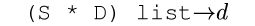. The compiler has a list of values and a list of variable names. It recurses down the lists, and emits a const and a cons instruction (making a binding) for each list member. At the base it recovers the terminator, then it returns up and emits cons instructions that build the spine. The copy function appears in Figure lce, and its specialization in Figure lcer.

It turns out that this lift compiler can be created by `cogen` itself. The meta-interpreter is just a structure-copy procedure that traverses the value and the binding times in parallel. A delayed lift annotation is used where the BTs indicate a simple lift. Specializing this to the binding times results in a copy function with lifts at the leaves. The value passed to the copy function has the binding time that was just a static value. When the continuation is finally called the remaining static information is propagated. The copy function may contain calls to itself (where the BT was circular) or to other extensions (to handle higher-order values).

This is an example of multi-stage compiler generation because the output of a generated compiler is being fed into `cogen`. The implementation requires care as `cogen` is being used to implement itself, but the possibility of the technique is encouraging.

```(define (copy_sdl2d l)
(if (null? l)
'()
(let ((hd (car l))
(tl (cdr l)))
(cons (cons (lift (car hd))
(cdr hd))
(copy_sdl2d tl)))))
```

Figure lce: Copy-function with lifts at the leaves. It is generated by the lift compiler to perform a lift from `(S * D) list` to `D`.

```sdl2d1(k a b) {
(car k)(k (cons (cons a 1) (cons (cons b 2) '())))
}```

Figure lcer: Result of specializing copy function.

#### Controlling Cyclic Values

`Set-base` is an special annotation that takes an integer with any binding time and converts it to cyclic with a particular base. This can be a lift, for example when going from static to cyclic base four, or when going from cyclic base four to base two. Note that cyclic base one is functionally the same as dynamic. Converting from dynamic to cyclic requires case-analysis (known as The Trick in partial evaluation jargon). Changing the base of a cyclic value may require both of these, for example to go from base two to base three.

Lifting out a factor is easy because static information is just discarded from the compiler. Case-analysis creates static information by emitting dynamic conditionals and duplicating code. Nitrous implements this by inserting a call to the procedure in Figure fdts. The argument `d` is the dynamic value to be converted; `n` is its maximum possible value plus one; `n` is static. Note that the test for the error is essential even though it may never be true, as without it the compiler will not terminate.

```finite-dynamic-to-static(k d n) {
if (zero? n) error
n1 = n - 1
if (n1 = d) \$inline = #t
(car k)(k n1)
\$inline = #t
finite-dynamic-to-static(k d n1)
}```

Figure fdts: Convert a dynamic value to static by case analysis.

### Details and Complications

#### Special Primitive Functions

`Cogen` treats some primitive functions specially, generally in order to preserve partially static information. Figure special-ops gives the improved binding times possible, and in what situations they occur. Notes:

a
implement copy propagation by just copying the shape.
b
because `root` is untyped.
c
for variable splitting.

d
the result is metastatic if the pair has never been joined with an atom (see Section bt). `Null?` and `atom?` are also supported.

e
`apply` takes two arguments: a primitive (in one back-end a C function, in the other a Scheme procedure) and a list of arguments. If the primitive and the number of arguments are static, then the compiler can just generate the primitive instead of building an argument list and generating an `apply`. This supports interpreters with an open set of primitives or a foreign function interface. Notice this does not improve the binding times, it just generates better code.

f
extensions for both `S` and `D` are created, the compiler chooses one statically (see Section cyclic).

g
`early=` conservative static equality of dynamic values, see Section loads.

```(identity x)x a (cons S S)Sb (cons S D)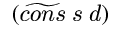(cons D D)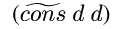c (car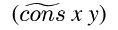)x (pair?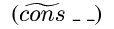)Sd (apply S (D list))De (+ C S)C (* C S)C (+ D S)C (* D S)C (zero? C)S Df (imod C S)S (idiv C S)C (early= D D)Sg ```

Figure special-ops: See the text for notes.

### Static Extensions

Previously [Draves96], the code pointer in a static closure (from a function or a continuation) was represented with a metastatic extension. The binding times in such an extension are fixed, which restricted the use of polyvariance and required additional annotation. Specifically, when you made a static stack frame you had to give the binding time of the return value. This conflicts with the polyvariance in the binding times needed to support bit-addressing.

Furthermore, the implementation of late normalization of cyclic values (Section npi) requires being able to identify all cyclic values in the static state of the compiler.

I solved these together by changing the representation of extension to include the original code pointer and the binding time of the first argument (i.e. the frame inside the closure). So when the compiler is running and the static extension is called, the rest of the binding times are available. At this point we call cogen to get the metastatic extension, and then jump to that. Because we memoize on binding times, this results in lazy compiler generation; cogen is called at compile time. In this way, Nitrous is a hybrid between offline and online systems like [Sperber96].

This was a big change conceptually, but a small change in terms of the code and what actually happens.

```
```

There are two places where static extensions are created: static recursions and higher-order values.

Because the stack is an explicit argument, when `cogen` encounters a static recursion the same label will eventually appear on two stack frames. In theory, because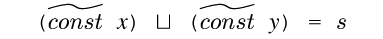, when this loop is collapsed the metastatic continuations would be lifted to static, thereby converted to extensions and forming a control stack in the compiler[footnote: If instead of forgetting x and y completely, we approximated them with a set, the result would be control-flow analysis based on abstract interpretation [Shivers91].]. However, to simplify the implementation `cogen` uses a special lift that makes a static extension: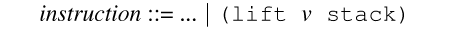This saves v and its binding time in a static extension, and sets its binding time to stack-bt.

For example, consider specialization of `append`[footnote: Only a irregular recursion really requires this, but `append` is easier to understand.]. The source with annotations appears in Figure stack-lift. When `cogen` is called to create ```append(D S D)```, it calls itself recursively to create ```append(stack-bt S D)```, causing a recursive call to create `append(stack-bt S D)` again. Since this binding-time pattern is now in the memo-table, the recursion terminates. There are two extensions made from `append`. The difference is the jump to the continuation in the base case. In the first the jump target is dynamic, in the second it is static (but not constant). If the list is not zero length then when we call `append(stack-bt S D)` with the static values, then each time the compiler returns it is a call to a static extension. The first return creates `cont((cont (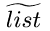stack-bt S D)) D)`. Subsequent returns except for the last one request the same binding-time pattern, which is in the memo-table. Figure slt illustrates this series of events. Note that there are two versions of each extension. `Cogen` could avoid producing the (probably over-) specialized entry/exit code by checking for the end of the stack explicitly. Static extensions are also created for source lambda abstractions, see the case `lambda?` in Appendix sal-src.

```
```

Late normalization of cyclic values requires that all cyclic values be identified and simultaneously normalized at the beginning of the construction of a dynamic code block (after checking the memotable). These values are identified by their binding times. So we need the binding times of the values inside of closures and the stack. This is the other reason.

```append(k l m) {
if (null? l) (car k)(k m)
frame = (list k l m)
cl = (close cont frame)
lift cl stack
append(cl (cdr l) m)
}
cont(self r) {
(k l m) = (cdr self)
nr = (cons (car l) r)
lift nr
(car k)(k nr)
}```

Figure stack-lift: Annotated code for a static recursion.

```append(D S D)
append(stack-bt S D)
*append(stack-bt S D)

cont((cont (stack-bt S D)) D)
*cont((cont (stack-bt S D)) D)
*cont((cont (stack-bt S D)) D)
...
cont((cont (D S D)) D)
```

Figure slt: Sequence of events when building a static recursion. A `*` indicates a hit on the memo-table.

#### Shape un/lifting and Sharing

Here we consider lift case 5 from Section lifting in greater detail. Say `f` has one argument besides itself. Then lifting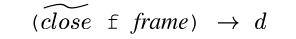creates a call to the extension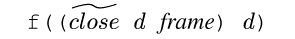. The extension is used to fold the static part of frame into `f`. The problem is, according to its binding-time pattern, the extension expects the dynamic part of the frame to be passed in separate registers (because of variable splitting), but at the call site the value is pure dynamic, so they are all stored in one register.

Nitrous uses special code at the call site to save (lift) the shape, and in the extension wrapper to restore (unlift) the shape. This code optimizes the transfer by only saving each register once, even if it appears several times in the shape (typically a lexical environment appears many times, but we only need to save the subject-values once). The same optimization prevents a normal jump from passing the same register more than once.

#### Dynamic Control Stacks

How do we extract the dynamic stack of a Sal program from the stack in the Sal interpreter? Say `cogen` encounters `do-call(stack-bt S S alist-bt)` (see Figure do-call). When `cl` is lifted we compute ```cont((S (stack-bt S)) D)```. We want to generate a procedure call where `cont` jumps to `apply`, so inlining is disabled and we lift the stack (`k`) to `D`, invoking lift base case 4. The problem is the extension was made assuming the code pointer would be static, but now it will be dynamic. The unlift code inserts an additional cdr to skip the dynamic value, thus allowing an irregular stack pattern to be handled.

```do-call(k fn exp env) {
frame = (list k fn)
cl = (close cont frame)
lift cl stack
eval(cl exp env)  a
}

cont(self arg) {
(k fn) = (cdr self)
lift k
\$inline = #f
apply(k fn arg)
}```

Figure do-call: annotated code to produce a dynamic stack frame. Notes: a the call to evaluate the argument is inlined.

#### Representations of Cyclic Values

If cyclic values as described in Sections cyclic are applied to the filter example from Chapter 5, a limitation is revealed. The problem is the addresses are cyclic values so before you can load a word the address must be lifted, resulting in a dynamic multiplication and addition. One way to solve this is to use a different representation: rather than use q as the dynamic value, one can use bq. This is premultiplication. On most RISC architectures the remaining addition can be folded into the load instruction.

The disadvantage of premultiplication is that multiplication and division can no longer maintain sharing information. Which representation is best depends on how the value is used. A constraint system should suffice to pick the correct representation.

## Sal

The name ``Sal'' is derived from ``sample language''. There are two versions of Sal, one in Nitrous, and is the input to the Simple system. This section concerns the former. After giving the syntax of the language, this section describes its implementation with Nitrous. Sal is basically a recursive equations language with threaded store, `let`, `lambda`, syntactic sugar, and annotations (that is, information ignored by the ordinary semantics of the language, but essential to `cogen`). Figure sal-syntax gives a grammar for the syntax. The semantics are obvious except as noted:

a
first-order call or primitive application, the name refers to a definition.
b
higher-order call, the first expression must evaluate to a lambda.
c
d
generates an assignment to the special `\$inline` variable.

See Appendix sal-src for the source code for the interpreter. That this is `root` code makes it difficult to read; if written in direct-style with high-level syntax, it would be ordinary. It could easily be bootstrapped and implemented in Sal itself.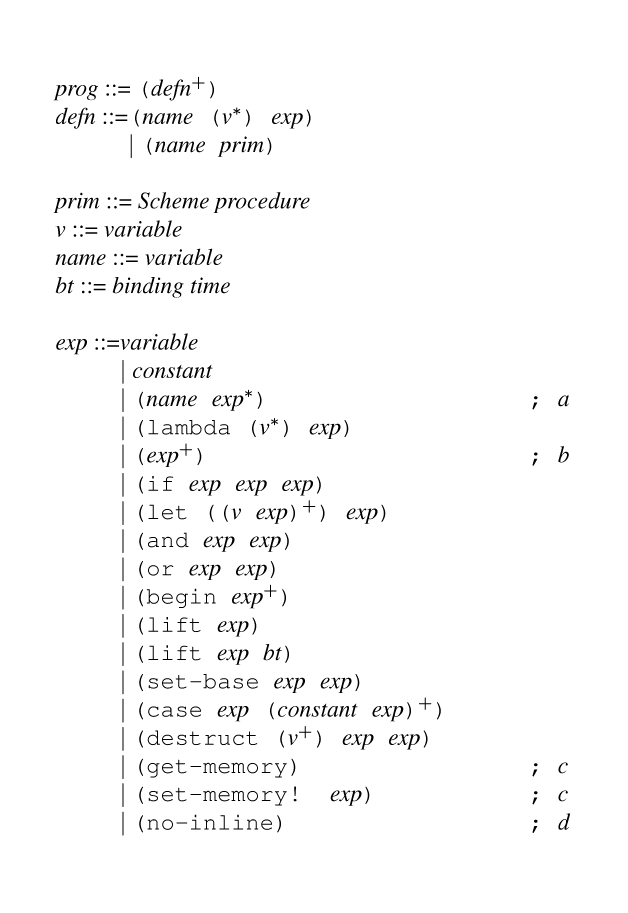Figure sal-syntax: The grammar for the syntax of Sal. See text for notes.

The compiler generated by `cogen` compiles Sal programs to `root` programs. It works in one-pass, performing CPS conversion, closure conversion using a linked representation, and executes tail-recursion without the stack. It generates some duplicate code unnecessarily, and is non-optimizing. Conversion to continuation-passing style is a standard result in PE [Danvy96], but the others are new.

It is possible to feed this generated code back into `cogen`. This is how the benchmarks of Section nitrous were done.

Examples of residual `root` code generated by this compiler appears in Appendex sal-obj. They show the duplication problems, as well as the residual annotations required for two-level specialization.